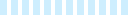## Parameters Overview### Default Measurement Units

The default measurement units in the Parameter table are Meters/Radians/Kilograms.
To change the measurement unit, simply type the unit you would like to use after the number (For example 20 mm or 30 deg).
This is important to note if you are seeing a number very different to expected, it could be caused by expecting the number to be in Millimetres, when it is in Metres.

### Parameter Levels

Scene Scene level parameters are stored in the IronCAD Scene file. Note: these will not be included in the Assembly/Part if you drop it into a catalog. Generally Assembly level Parameters are much better to use.

Assembly Assembly level parameters are stored at the Assembly level.

Pattern Pattern level parameters are stored at the Pattern level.

Part Part level parameters are stored at the Part level.

Shape Shape level parameters are stored at the Intellishape/Shape level.

Profile Profile level parameters are stored at the 2D sketch Profile level.

### Basic Parameter Functions and Options

X+Y  X plus Y

X-Y X minus Y

X*Y X multiplied by Y

X/Y X divided by Y

PI() The constant π (3.14159...)

ABS(X) Absolute value of X.

SIN(X) Sine of X, where X is an angle in radians (type "deg" for Degrees).

COS(X) Cosine of X, where X is an angle in radians (type "deg" for Degrees).

TAN(X) Tangent of X, where X is an angle in radians (type "deg" for Degrees).

ASIN(X) Arcsine of X in the range -π/2 to π/r radians. If X is less than -1 or greater than 1, ASIN returns an indefinite value.

ACOS(X) Arccosine of X in the range 0 to π/2 radians. If X is less than -1 or greater than 1 ACOS returns an indefinite value.

ATAN(X) Arctangent of X. If X is 0, ATAN returns 0. ATAN returns a value in the range -π/2 to π/2 radians.

ATAN2(Y,X) Arctangent of y/x. If both parameters are 0, the function returns 0. ATAN2 returns a value in the range -π to π radians, using the signs of both parameters to determine the quadrant of the return value.

SQRT(X) Square roor of X. If X < 0, the evaluation fails and the value of the variable is unchanged.

SQR(X) Square of X.

POW(X,Y) X to the power of Y.

IF (X,Y,Z) If evaluates X. If X evaluates to TRUE (non-zero), Y is returned, else Z is returned.

MAX (X,Y,...Z) MAX evaluates all arguments and returns the largest value. MAX takes a variable number of arguments.

MIN (X,Y,...Z) MIN evaluates all arguments and returns the smallest value. MIN takes a variable number of arguments.

INT(X) INT evaluates X and returns the integer parts of the evaluation result.

SWITCH(X, G1, V1, G2, V2, ......., Gn, Vn, Vdef) SWITCH returns V1 if the evaluation results of X is equal to the evaluation result of G1, otherwise returns V2 if the evaluation result of X is equal to the evaluation result of G2, otherwise returns V3 if the evaluation results of X is equal to the evaluation results of G3. otherwise returns V4 is the evaluation result of X is equal to the evaluation result of G4, .....(so on)...... otherwise returns Vn if the evaluation result of X is equal to the evaluation result of Gn, otherwise returns Vdef. For example, SWITCH (X, 5, 10, 6, 12, 8) returns 10 if X is equal to 5. It returns 12 if X is equal to 6. If X is neither 5 nor 6, it returns 8.

SUPPRESSION   |   The suppression Parameter is a different type of Parameter, used to suppress items. This supports only 1 and 0 values. 1 = suppressed, 0 = unsuppressed. Note this Parameter type is selected via the dropdown and cannot be typed like a standard function.

#### Getting Sizebox Values to Drive a Parameter

You can control/drive a parameter with Sizebox values of an intellishape, part, or assembly. To do this, type Sizebox\Length or Sizebox\Width or Sizebox\Height. You can also get the Sizebox value from a parent item, my typing "Parent\" at the beginning. For example, Parent\Sizebox\Length or Parent\Parent\Parent\Sizebox\Length. Note these are backslashes not forward slashes.

#### Reserved Parameter Names

Some names are reserved for system parameters and expressions. You will not be able to name any of your parameters with a reserved name. Reserved names include Funtions, Units, XYZ, and more. Here is a list: PI, SQR, VALTOSTR, VEC, CONST, ABS, IF, CELL, NORM, IN, SIN, MAX, FILLETPVALUE, DOT, FT, COS, MIN, FACE, CROSS, YD, TAN, XFORM, ENTITY, PERP, MI, ASIN, IXFORM, PAR, X, MM, ACOS, FRAME, SOLVE, Y, CM, ATAN, PT, PLANE, Z, M, ATAN2, DIR, ROTW, POS, SQRT, PTPTDIST, NOTIFY, GUARD, INT, SWITCH, DEG, RAD.